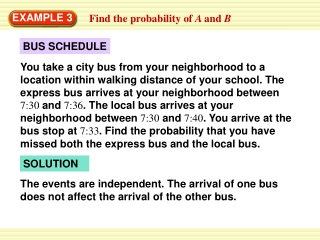DownloadDownload PresentationEXAMPLE 3

# EXAMPLE 3

Télécharger la présentation## EXAMPLE 3

- - - - - - - - - - - - - - - - - - - - - - - - - - - E N D - - - - - - - - - - - - - - - - - - - - - - - - - - -
##### Presentation Transcript

1. EXAMPLE 3 Find the probability of A and B BUS SCHEDULE You take a city bus from your neighborhood to a location within walking distance of your school. The express bus arrives at your neighborhood between 7:30 and 7:36. The local bus arrives at your neighborhood between 7:30 and 7:40. You arrive at the bus stop at 7:33. Find the probability that you have missed both the express bus and the local bus. SOLUTION The events are independent. The arrival of one bus does not affect the arrival of the other bus.

2. 1 2 3 10 = = = 3 6 EXAMPLE 3 Find the probability of A and B There are 6 minutes whenthe express bus can arrive.You are not at the bus stopfor 3 of those minutes. There are 10 minutes whenthe local bus can arrive.You are not at the bus stopfor 3 of those minutes. P(you miss express bus) P(you miss local bus)

3. 3 3 = = 10 20 ANSWER 1 2 The probability that you miss the express bus and the local bus is 3 20 EXAMPLE 3 Find the probability of A and B Multiply the probabilities of the two events: P(you miss both buses)

4. 2 7 3 8 P(blue and then blue) = P(blue)P(blue given blue) 3 6 = = = 56 28 EXAMPLE 4 Find the probability of A and B PEN COLORS A box contains 3 blue pens and 5 black pens. You choose one pen at random, do not replace it, then choose a second pen at random. What is the probability that both pens are blue? SOLUTION Because you do not replace the first pen, the events are dependent. Before you choose a pen, there are 8 pens, and 3 of them are blue. After you choose a blue pen, there are 7 pens left and 2 of them are blue.

5. 16 121 ANSWER 6 ANSWER 55 for Examples 3 and 4 GUIDED PRACTICE 3. MARBLES A bag contains 4 red, 5 green, and 2 blue marbles. You randomly draw 2 marbles, one at a time. Find the probability that both are red if: a. You replace the first marble. b. You do not replace the first marble.